UIHistories Project: A History of the University of Illinois by Kalev Leetaru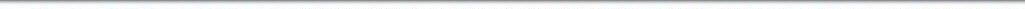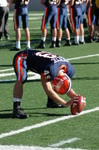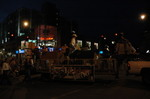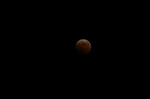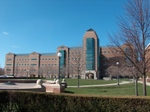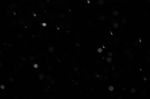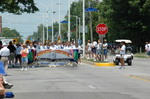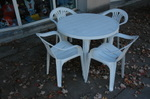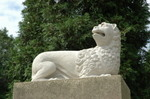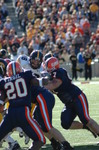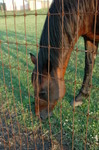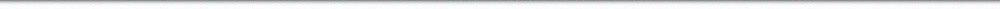N A V I G A T I O N D I G I T A L L I B R A R Y

## Repository: UIHistories Project: Board of Trustees Minutes - 1896 [PAGE 74]

Caption: Board of Trustees Minutes - 1896
This is a reduced-resolution page image for fast online browsing.

< Previous Page [Displaying Page 74 of 371] Next Page >
[VIEW ALL PAGE THUMBNAILS]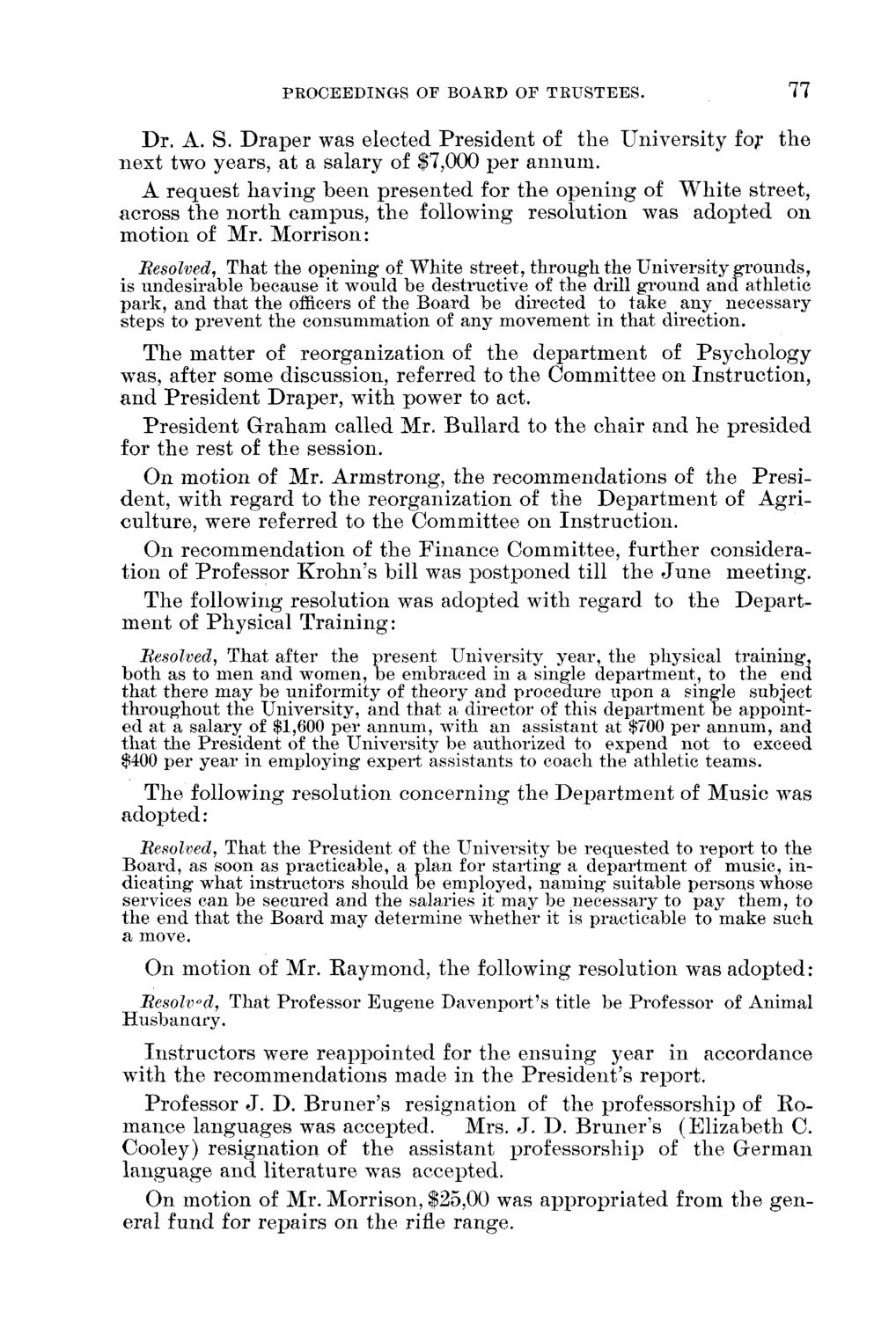### EXTRACTED TEXT FROM PAGE:

PROCEEDINGS OF BOARD OF TRUSTEES.

77

D r . A. S. D r a p e r was elected P r e s i d e n t of t h e U n i v e r s i t y for t h e n e x t two years, at a salary of \$7,000 p e r a n n u m . A r e q u e s t h a v i n g b e e n p r e s e n t e d for t h e o p e n i n g of W h i t e street, across t h e n o r t h c a m p u s , t h e following resolution was a d o p t e d on motion of Mr. M o r r i s o n : Resolved, That the opening of White street, through the University grounds, is undesirable because it would be destructive of the drill ground and athletic park, and that the officers of the Board be directed to take any necessary steps to prevent the consummation of any movement in that direction. T h e m a t t e r of r e o r g a n i z a t i o n of t h e d e p a r t m e n t of P s y c h o l o g y wTas, after some discussion, referred to t h e C o m m i t t e e on I n s t r u c t i o n , a n d P r e s i d e n t D r a p e r , with power to act. P r e s i d e n t G r a h a m called Mr. B u l l a r d to t h e chair a n d h e p r e s i d e d for t h e rest of t h e session. O n m o t i o n of Mr. A r m s t r o n g , t h e r e c o m m e n d a t i o n s of t h e P r e s i d e n t , with r e g a r d to t h e reorganization of t h e D e p a r t m e n t of A g r i c u l t u r e , were referred to t h e C o m m i t t e e on I n s t r u c t i o n . O n r e c o m m e n d a t i o n of t h e F i n a n c e Committee, further considerat i o n of Professor K r o h n ' s bill was p o s t p o n e d till t h e J u n e m e e t i n g . T h e following resolution was adopted with regard to t h e D e p a r t m e n t of P h y s i c a l T r a i n i n g : Resolved, That after the present University year, the physical training, both as to men and women, be embraced in a single department, to the end that there may be uniformity of theory and procedure upon a single subject throughout the University, and that a director of this department be appointed at a salary of \$1,600 per annum, with an assistant at \$700 per annum, and that the President of the University be authorized to expend not to exceed \$400 per year in employing expert assistants to coach the athletic teams. T h e following resolution c o n c e r n i n g t h e D e p a r t m e n t of M u s i c was adopted: Resolved, That the President of the University be requested to report to the Board, as soon as practicable, a plan for starting a department of music, indicating what instructors should be employed, naming suitable persons whose services can be secured and the salaries it may be necessary to pay them, to the end that the Board may determine whether it is practicable to make such a move. O n motion of Mr. R a y m o n d , t h e following resolution was a d o p t e d : Resolved, That Professor Eugene Davenport's title be Professor of Animal Husbandry. I n s t r u c t o r s were r e a p p o i n t e d for t h e e n s u i n g year in accordance with t h e r e c o m m e n d a t i o n s m a d e in t h e P r e s i d e n t ' s report. Professor J . D . B r u n e r ' s r e s i g n a t i o n of t h e professorship of R o m a n c e l a n g u a g e s was accepted. M r s . J . D . B r u n e r ' s ( E l i z a b e t h C. Cooley) r e s i g n a t i o n of t h e assistant professorship of t h e G e r m a n l a n g u a g e a n d l i t e r a t u r e was accepted. O n motion of Mr. Morrison, \$25,00 was a p p r o p r i a t e d from t h e g e n eral fund for r e p a i r s on t h e rifle r a n g e .